SNOS975G May   2001  – August 2015

PRODUCTION DATA.

1. 1Features
2. 2Applications
3. 3Description
4. 4Revision History
5. 5Pin Configuration and Functions
6. 6Specifications
7. 7Device and Documentation Support
8. 8Mechanical, Packaging, and Orderable Information

• DGK|8
• DGK|8

## 6 Specifications

### 6.1 Absolute Maximum Ratings (1)(2)

over operating free-air temperature range (unless otherwise noted) (6)
MIN MAX UNIT
VIN Differential +/−10 V
Output Short Circuit Duration See (3)(4)
Supply Voltage (V+ - V) 24 V
Voltage at Input/Output pins V+ +0.8, V −0.8 V
Junction Temperature (5) +150 °C
Storage temperature range, Tstg −65 +150 °C
Soldering Information:    Infrared or Convection (20 sec.) 235 °C
Wave Soldering (10 sec.) 260 °C
(1) Absolute Maximum Ratings indicate limits beyond which damage to the device may occur. Operating Rating indicate conditions for which the device is intended to be functional, but specific performance is not ensured. For ensured specifications and the test conditions, see the Electrical Characteristics.
(2) If Military/Aerospace specified devices are required, please contact the TI Sales Office/ Distributors for availability and specifications.
(3) Applies to both single‐supply and split‐supply operation. Continuous short circuit operation at elevated ambient temperature can result in exceeding the maximum allowed junction temperature of 150°C.
(4) Output short circuit duration is infinite for VS ≤ 6V at room temperature and below. For VS > 6V, allowable short circuit duration is 1.5ms.
(5) The maximum power dissipation is a function of TJ(max), RθJA, and TA. The maximum allowable power dissipation at any ambient temperature is PD = (TJ(max) - TA)/RθJA. All numbers apply for packages soldered directly onto a PC board.
(6) Stresses beyond those listed under Absolute Maximum Ratings may cause permanent damage to the device. These are stress ratings only, which do not imply functional operation of the device at these or any other conditions beyond those indicated under Recommended Operating Conditions. Exposure to absolute-maximum-rated conditions for extended periods may affect device reliability.

### 6.2 ESD Ratings

VALUE UNIT
V(ESD) Electrostatic discharge(1) Human Body Model (HBM), per ANSI/ESDA/JEDEC JS-001, all pins(2) ±2000 V
Machine Model (MM)(3) ±200
(1) Human Body Model, 1.5 kΩ in series with 100 pF. Machine Model, 0 Ω is series with 200 pF.
(2) JEDEC document JEP155 states that 2000-V HBM allows safe manufacturing with a standard ESD control process.
(3) JEDEC document JEP157 states that 200-V MM allows safe manufacturing with a standard ESD control process.

### 6.3 Recommended Operating Conditions

over operating free-air temperature range (unless otherwise noted)
MIN MAX UNIT
Supply Voltage (V+ - V) 2.5 22 V
Operating Temperature Range(5) −40 +85 °C

### 6.4 Thermal Information

THERMAL METRIC(1) DGK UNIT
8 PINS
RθJA Junction-to-ambient thermal resistance(2) 235 °C/W
(2) The maximum power dissipation is a function of TJ(max),RθJA, and TA. The maximum allowable power dissipation at any ambient temperature is PD = (TJ(max) - TA)/RθJA. All numbers apply for packages soldered directly onto a PC board.

### 6.5 2.7V Electrical Characteristics

Unless otherwise specified, all limits ensured for V+ = 2.7V, V = 0V, VCM = 0.5V, VO = V+/2, and RL > 1MΩ to V. Boldface limits apply at the temperature extremes.
PARAMETER TEST CONDITIONS MIN(2) TYP(1) MAX(2) UNIT
VOS Input Offset Voltage VCM = 0.5V & VCM = 2.2V +/−0.7 +/−5
+/−7
mV
TC VOS Input Offset Average Drift VCM = 0.5V & VCM = 2.2V
(3)
+/−2 µV/C
IB Input Bias Current VCM = 0.5V
(4)
−1.20 −2.00
−2.70
µA
VCM = 2.2V
(4)
+0.49 +1.00
+1.60
IOS Input Offset Current VCM = 0.5V & VCM = 2.2V 20 250
400
nA
CMRR Common Mode Rejection Ratio VCM stepped from 0V to 1.0V 76
60
100 dB
VCM stepped from 1.7V to 2.7V 100
VCM stepped from 0V to 2.7V 58
50
70
+PSRR Positive Power Supply Rejection Ratio V+ = 2.7V to 5V 78
74
104 dB
CMVR Input Common-Mode Voltage Range CMRR > 50dB −0.3 −0.1
0.0
V
2.8
2.7
3.0 V
AVOL Large Signal Voltage Gain VO = 0.5 to 2.2V,
RL = 10k to V
70
67
78 dB
VO = 0.5 to 2.2V,
RL = 2k to V
67
63
73 dB
VO Output Swing
High
RL = 10k to V 2.49
2.46
2.59 V
RL = 2k to V 2.45
2.41
2.53
Output Swing
Low
RL = 10k to V 90 100
120
mV
ISC Output Short Circuit Current Sourcing to V
VID = 200mV (5)(6)
30
20
48 mA
Sinking to V+
VID = −200mV (5)(6)
50
30
65
IS Supply Current (both amps) No load, VCM = 0.5V 2.0 2.5
3.0
mA
SR Slew Rate (7) AV = +1,VI = 2VPP 9 V/µs
fu Unity Gain-Frequency VI = 10mV, RL = 2kΩ to V+/2 10 MHz
GBWP Gain Bandwidth Product f = 50KHz 15.5
14
21 MHz
Phim Phase Margin VI = 10mV 50 Deg
en Input-Referred Voltage Noise f = 2KHz, RS = 50Ω 15 nV/ √Hz
in Input-Referred Current Noise f = 2KHz 1 pA/ √Hz
fmax Full Power Bandwidth ZL = (20pF || 10kΩ) to V+/2 1 MHz
(1) Typical Values represent the most likely parametric norm.
(2) All limits are ensured by testing or statistical analysis.
(3) Offset voltage average drift determined by dividing the change in VOS at temperature extremes into the total temperature change.
(4) Positive current corresponds to current flowing into the device.
(5) Short circuit test is a momentary test.
(6) Output short circuit duration is infinite for VS ≤ 6V at room temperature and below. For VS > 6V, allowable short circuit duration is 1.5ms.
(7) Slew rate is the slower of the rising and falling slew rates. Connected as a Voltage Follower.

### 6.6 5V Electrical Characteristics

Unless otherwise specified, all limits ensured for V+ = 5V, V = 0V, VCM = 1V, VO = V+/2, and RL > 1MΩ to V. Boldface limits apply at the temperature extremes.
PARAMETER TEST CONDITIONS MIN(2) TYP(1) MAX(2) UNIT
VOS Input Offset Voltage VCM = 1V & VCM = 4.5V +/−0.7 +/−5
+/− 7
mV
TC VOS Input Offset Average Drift VCM = 1V & VCM = 4.5V
(3)
+/−2 µV/°C
IB Input Bias Current VCM = 1V
(4)
−1.18 −2.00
2.70
µA
VCM = 4.5V
(4)
+0.49 +1.00
+1.60
IOS Input Offset Current VCM = 1V & VCM = 4.5V 20 250
400
nA
CMRR Common Mode Rejection Ratio VCM stepped from 0V to 3.3V 84
72
110 dB
VCM stepped from 4V to 5V 100
VCM stepped from 0V to 5V 64
61
80
+PSRR Positive Power Supply Rejection Ratio V+ = 2.7V to 5V, VCM = 0.5V 78
74
104 dB
CMVR Input Common-Mode Voltage Range CMRR > 50dB −0.3 −0.1
0.0
V
5.1
5.0
5.3 V
AVOL Large Signal Voltage Gain VO = 0.5 to 4.5V,
RL = 10k to V
74
70
84 dB
VO = 0.5 to 4.5V,
RL = 2k to V
70
66
80
VO Output Swing
High
RL = 10k to V 4.75
4.72
4.87 V
RL = 2k to V 4.70
4.66
4.81
Output Swing
Low
RL = 10k to V 86 125
135
mV
ISC Output Short Circuit Current Sourcing to V
VID = 200mV (5)(6)
35
20
53 mA
Sinking to V+
VID = −200mV (5)(6)
60
50
75
IS Supply Current (both amps) No load, VCM = 1V 2.3 2.8
3.5
mA
SR Slew Rate (7) AV = +1, VI = 5VPP 10
7
12 V/µs
fu Unity Gain Frequency VI = 10mV,
RL = 2kΩ to V+/2
10.5 MHz
GBWP Gain-Bandwidth Product f = 50KHz 16
15
21 MHz
Phim Phase Margin VI = 10mV 53 Deg
en Input-Referred Voltage Noise f = 2KHz, RS = 50Ω 15 nV/ √Hz
in Input-Referred Current Noise f = 2KHz 1 pA/ √Hz
fmax Full Power Bandwidth ZL = (20pF || 10kΩ) to V+/2 900 KHz
tS Settling Time (+/−5%) 100mVPP Step, 500pF load 400 ns
THD+N Total Harmonic Distortion + Noise RL = 1kΩ to V+/2
f = 10KHz to AV= +2, 4VPP swing
0.05%
(1) Typical Values represent the most likely parametric norm.
(2) All limits are ensured by testing or statistical analysis.
(3) Offset voltage average drift determined by dividing the change in VOS at temperature extremes into the total temperature change.
(4) Positive current corresponds to current flowing into the device.
(5) Short circuit test is a momentary test.
(6) Output short circuit duration is infinite for VS ≤ 6V at room temperature and below. For VS > 6V, allowable short circuit duration is 1.5ms.
(7) Slew rate is the slower of the rising and falling slew rates. Connected as a Voltage Follower.

### 6.7 +/−11V Electrical Characteristics

Unless otherwise specified, all limits ensured for V+ = 11V, V = −11V, VCM = 0V, VO = 0V, and RL > 1MΩ to 0V. Boldface limits apply at the temperature extremes.
PARAMETER TEST CONDITIONS MIN(2) TYP(1) MAX(2) UNIT
VOS Input Offset Voltage VCM = −10.5V & VCM = 10.5V +/−0.7 +/−7
+/− 9
mV
TC VOS Input Offset Average Drift VCM = −10.5V & VCM = 10.5V
(7)
+/−2 µV/°C
IB Input Bias Current VCM = −10.5V
(3)
−1.05 −2.00
−2.80
µA
VCM = 10.5V
(3)
+0.49 +1.00
+1.50
IOS Input Offset Current VCM = −10.5V & VCM = 10.5V 30 275
550
nA
CMRR Common Mode Rejection Ratio VCM stepped from −11V to 9V 84
80
100 dB
VCM stepped from 10V to 11V 100
VCM stepped from −11V to 11V 74
72
88
+PSRR Positive Power Supply Rejection Ratio V+ = 9V to 11V 70
66
100 dB
−PSRR Negative Power Supply Rejection Ratio V = −9V to −11V 70
66
100 dB
CMVR Input Common-Mode Voltage Range CMRR > 50dB −11.3 −11.1
−11.0
V
11.1
11.0
11.3 V
AVOL Large Signal Voltage Gain VO = 0V to +/−9V,
RL = 10kΩ
78
74
85 dB
VO = 0V to +/−9V,
RL = 2kΩ
72
66
79
VO Output Swing
High
RL = 10kΩ 10.65
10.61
10.77 V
RL = 2kΩ 10.6
10.55
10.69
Output Swing
Low
RL = 10kΩ −10.98 −10.75
−10.65
V
RL = 2kΩ −10.91 −10.65
−10.6
ISC Output Short Circuit Current Sourcing to ground
VID = 200mV (5)(6)
40
25
60 mA
Sinking to ground
VID = 200mV (5)(6)
65
55
100
IS Supply Current No load, VCM = 0V 2.5 4
5
mA
SR Slew Rate
(4)
AV = +1, VI = 16VPP 10
8
15 V/µs
fU Unity Gain Frequency VI = 10mV, RL = 2kΩ 13 MHz
GBWP Gain-Bandwidth Product f = 50KHz 18
16
24 MHz
Phim Phase Margin VI = 10mV 58 Deg
en Input-Referred Voltage Noise f = 2KHz, RS = 50Ω 15 nV/ √Hz
in Input-Referred Current Noise f = 2KHz 1 pA/ √Hz
tS Settling Time (+/−1%, AV = +1) Positive Step, 5VPP 320 ns
Negative Step, 5VPP 600
THD+N Total Harmonic Distortion +Noise RL = 1kΩ, f = 10KHz,
AV = +2, 15VPP swing
0.01%
CTREJ Cross-Talk Rejection f = 5MHz, Driver
RL = 10kΩ
68 dB
(1) Typical Values represent the most likely parametric norm.
(2) All limits are ensured by testing or statistical analysis.
(3) Positive current corresponds to current flowing into the device.
(4) Slew rate is the slower of the rising and falling slew rates. Connected as a Voltage Follower.
(5) Short circuit test is a momentary test.
(6) Output short circuit duration is infinite for VS ≤ 6V at room temperature and below. For VS > 6V, allowable short circuit duration is 1.5ms.
(7) Offset voltage average drift determined by dividing the change in VOS at temperature extremes into the total temperature change.

### 6.8 Typical Performance Characteristics

TA = 25°C, Unless Otherwise Noted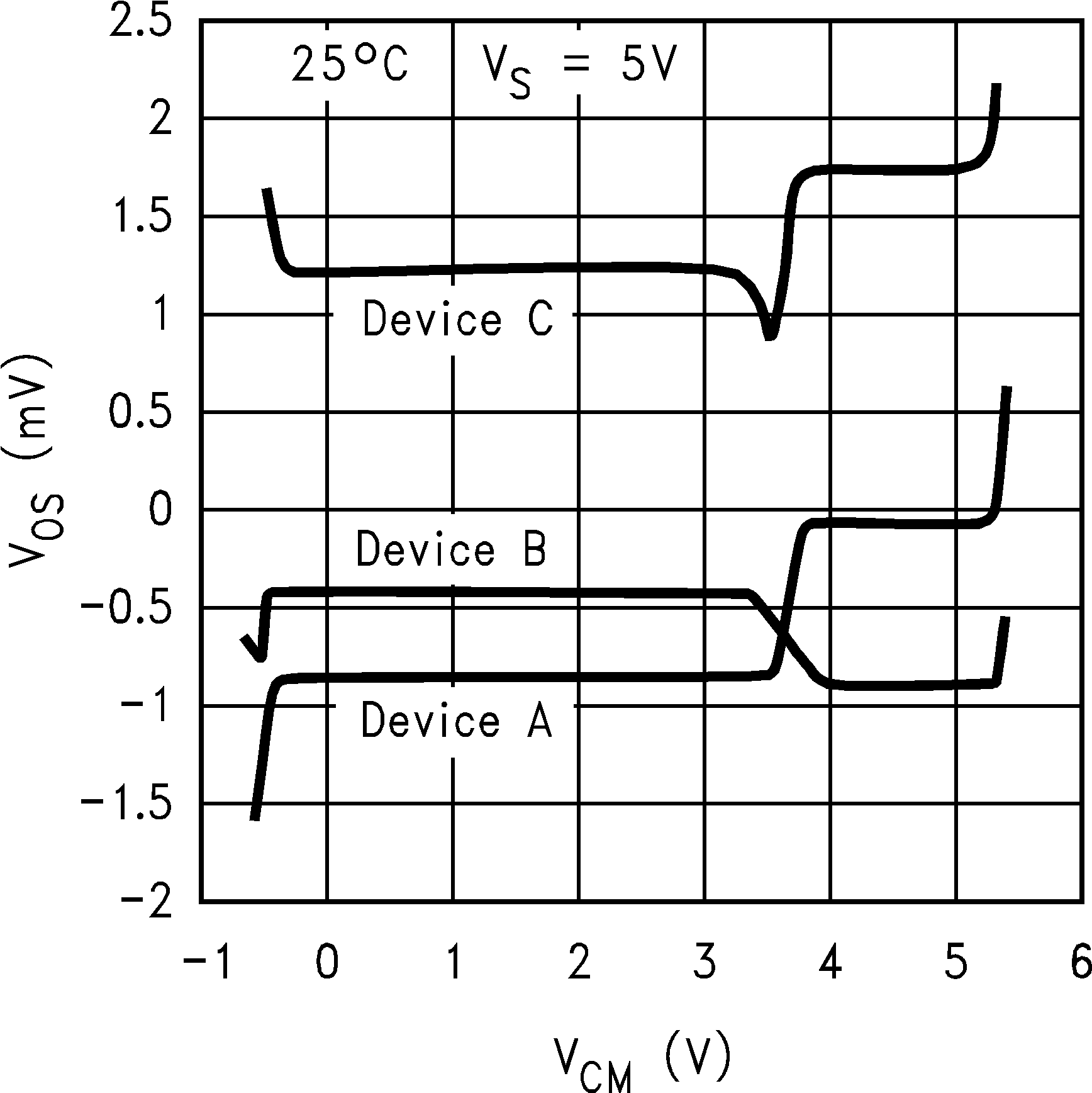Figure 1. VOS vs. VCM for 3 Representative Units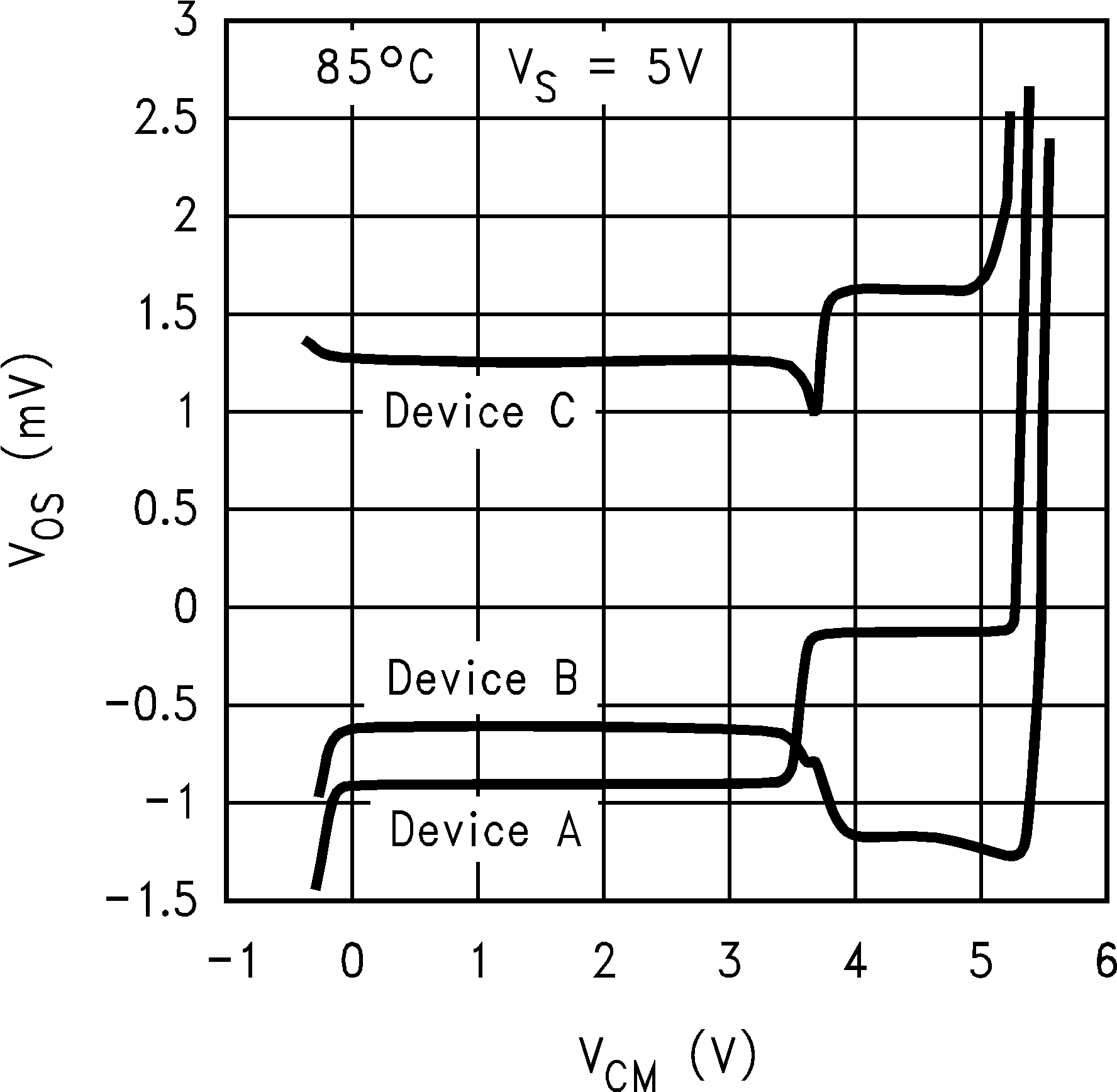Figure 3. VOS vs. VCM for 3 Representative Units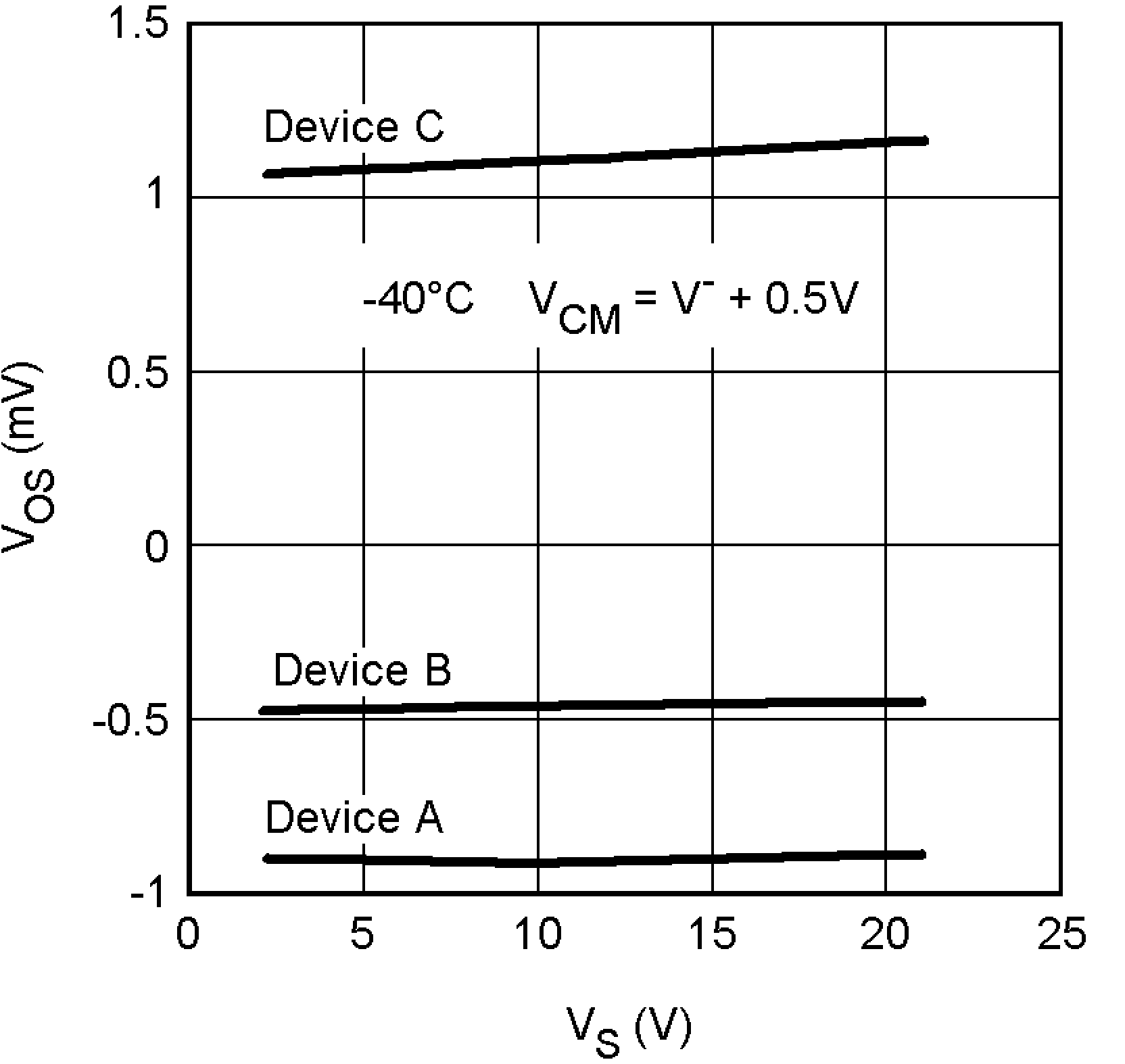Figure 5. VOS vs. VS for 3 Representative Units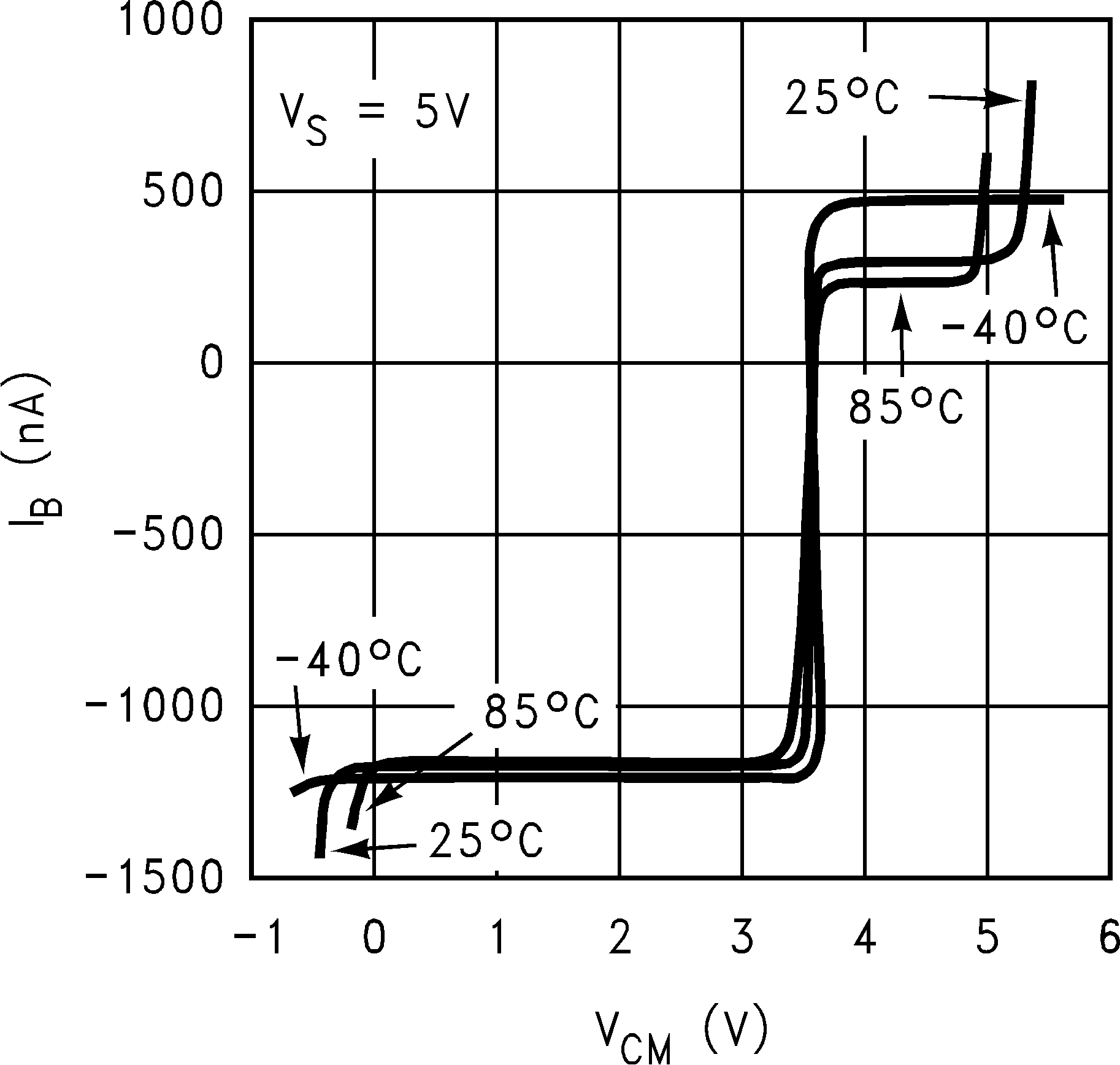Figure 7. IB vs. VCMFigure 9. IS vs. VCM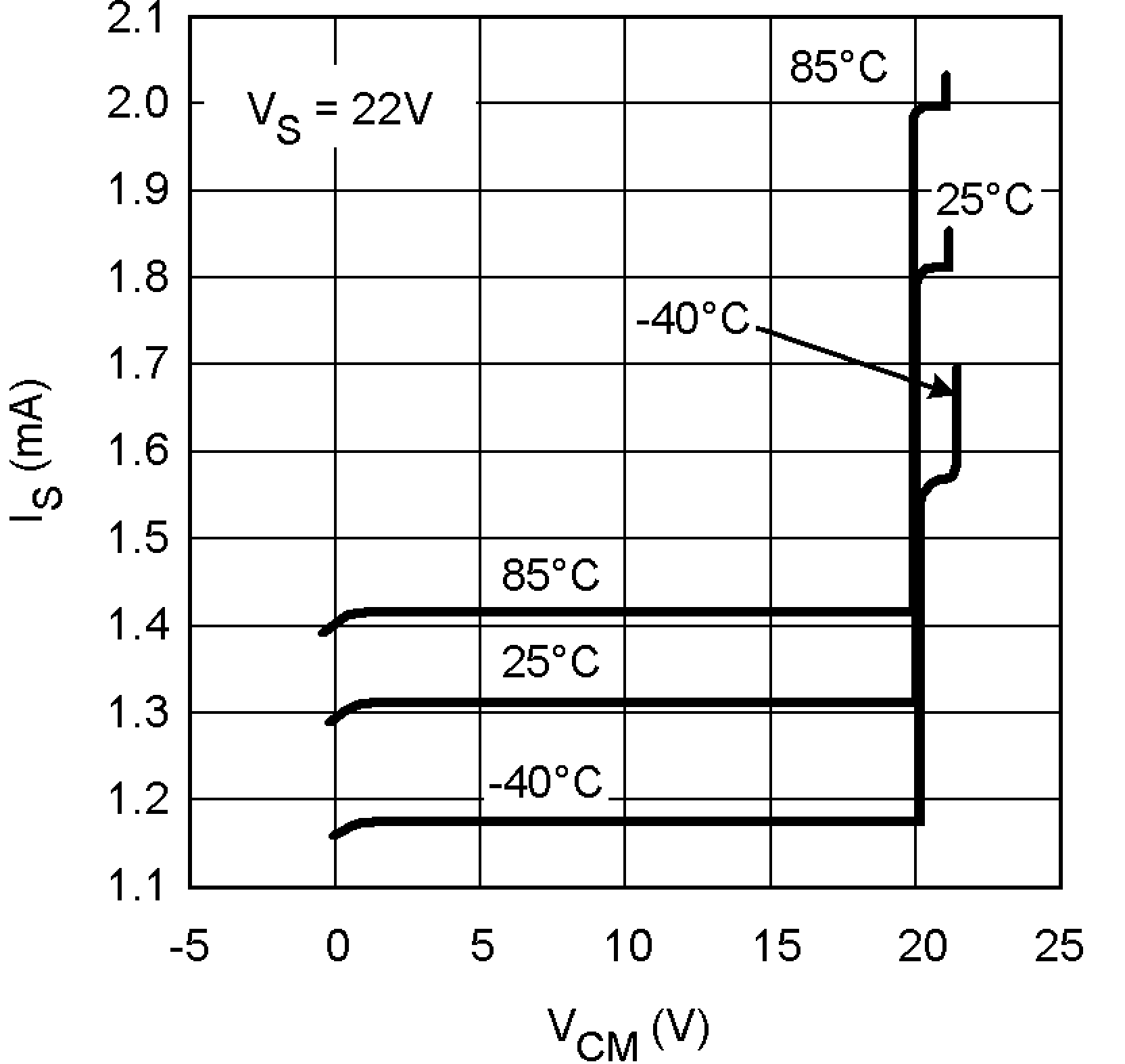Figure 11. IS vs. VCM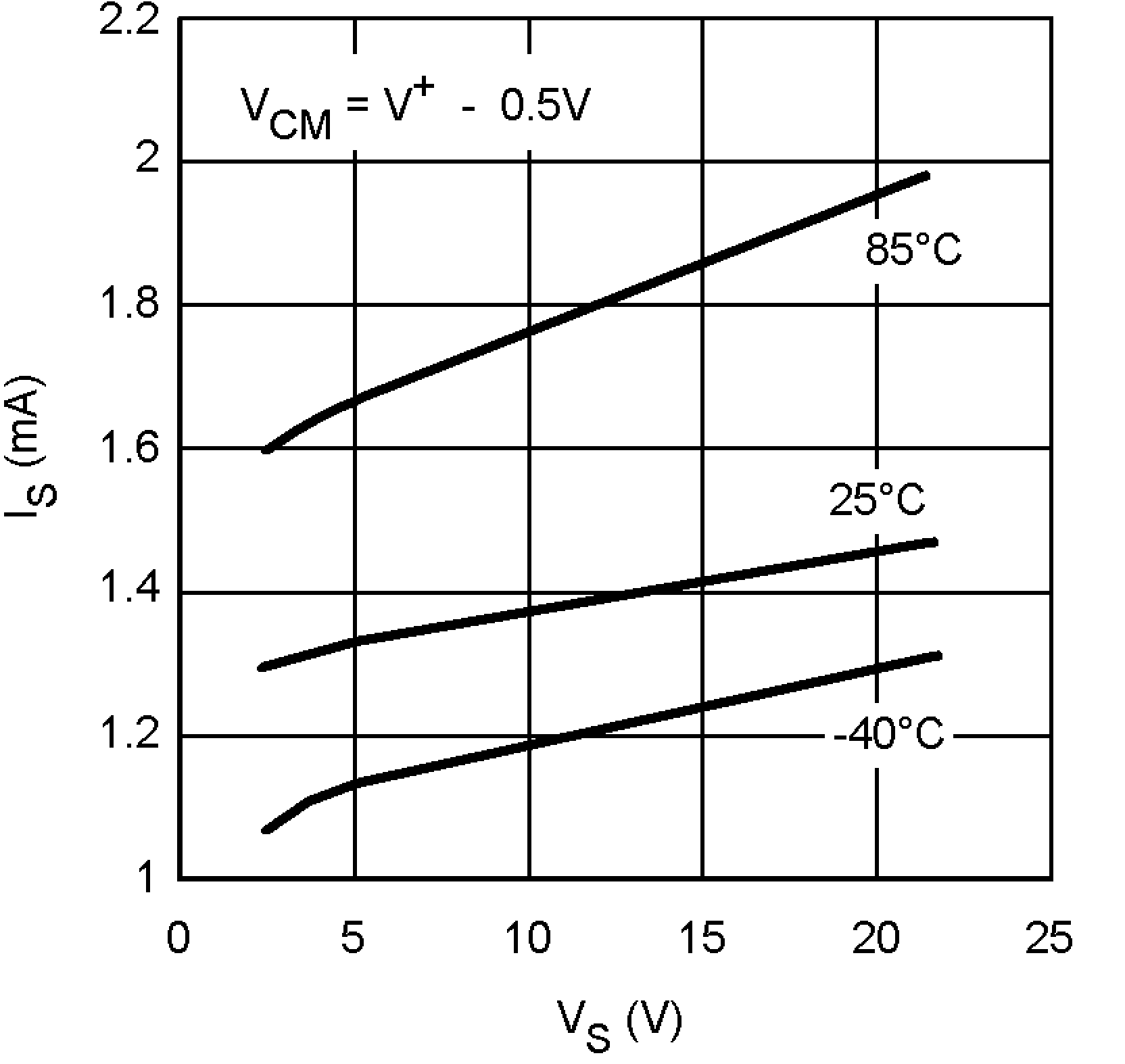Figure 13. IS vs. VS (NPN side)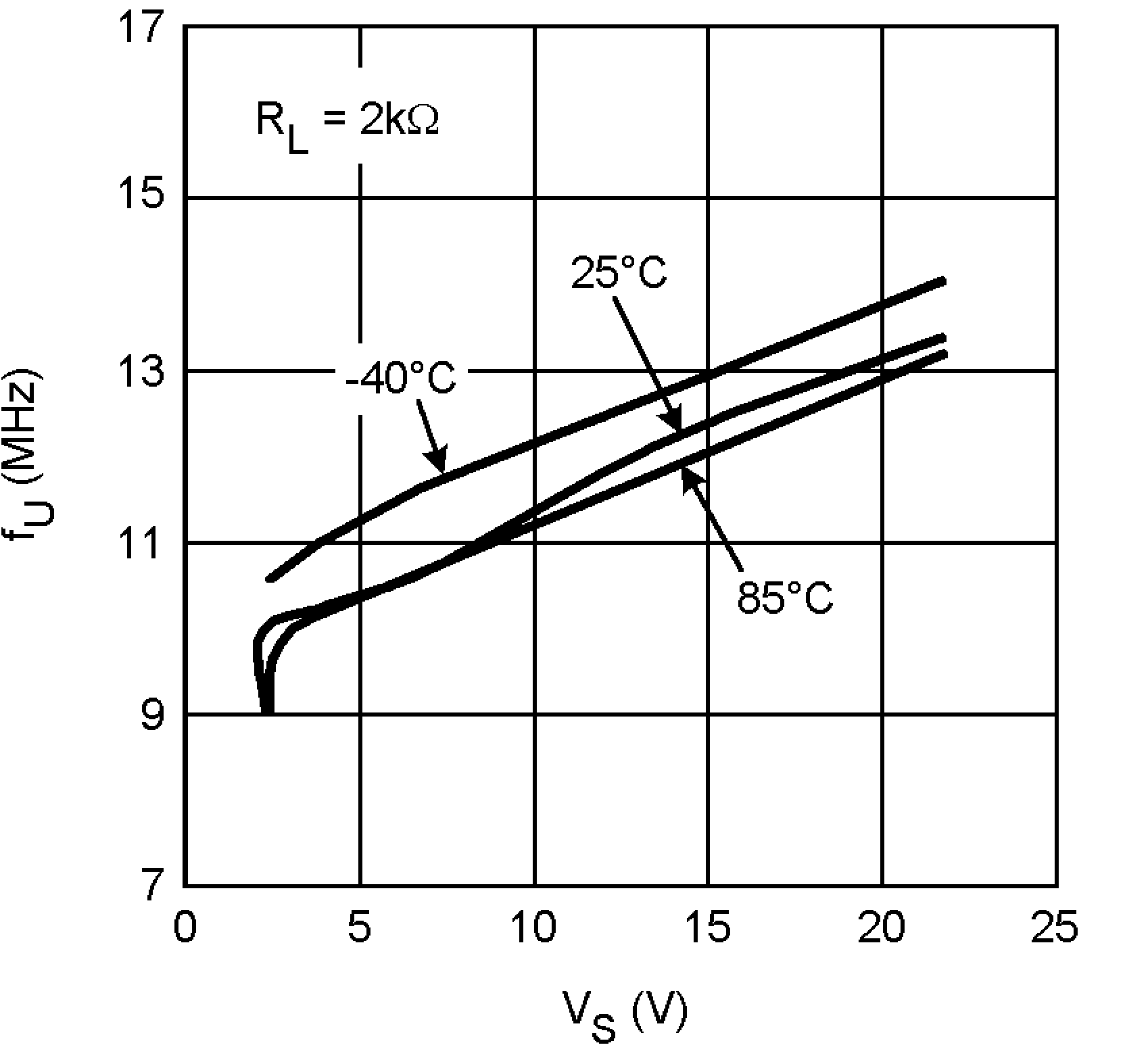Figure 15. Unity Gain Frequency vs. VS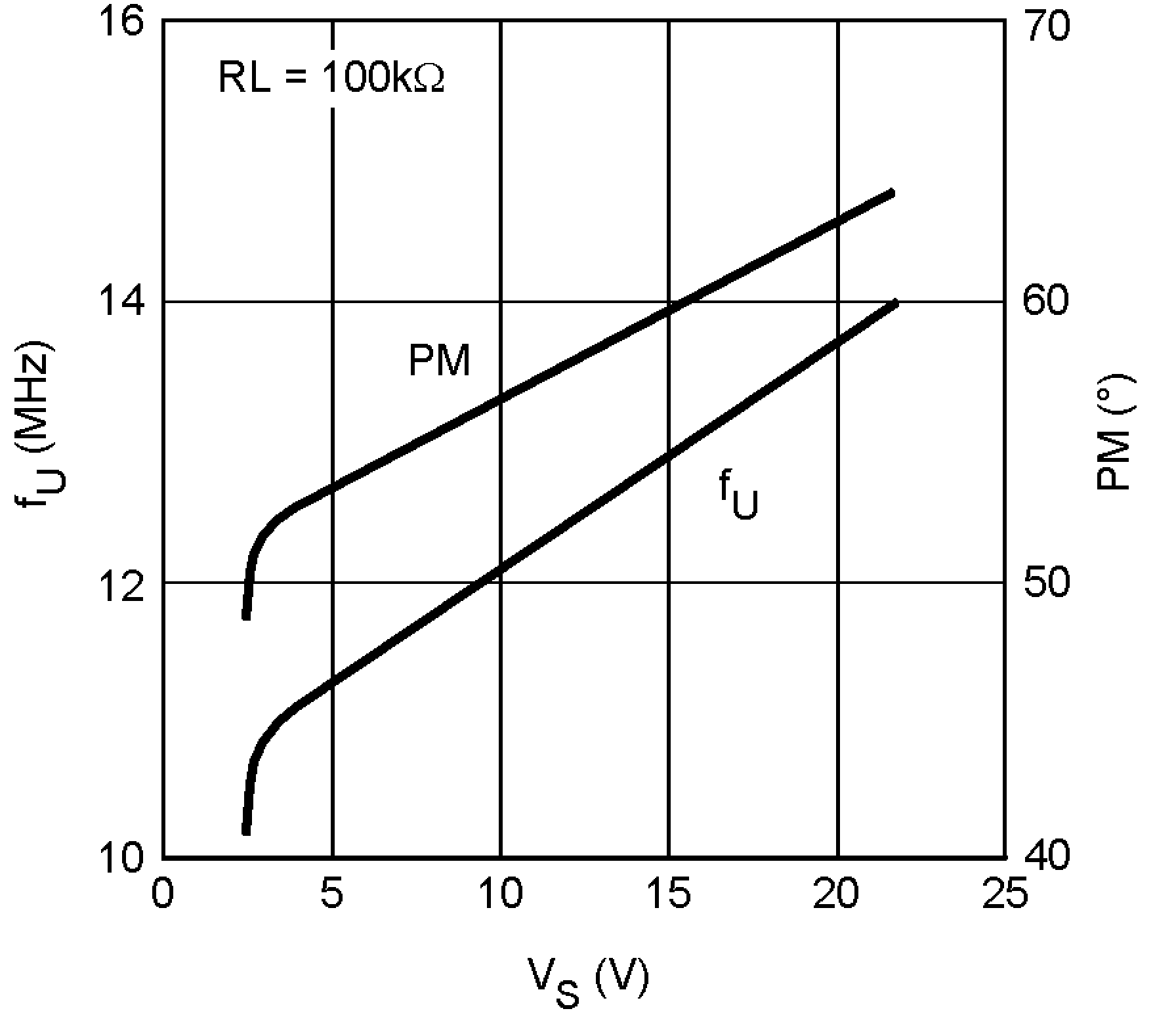Figure 17. Unity Gain Freq. and Phase Margin vs. VS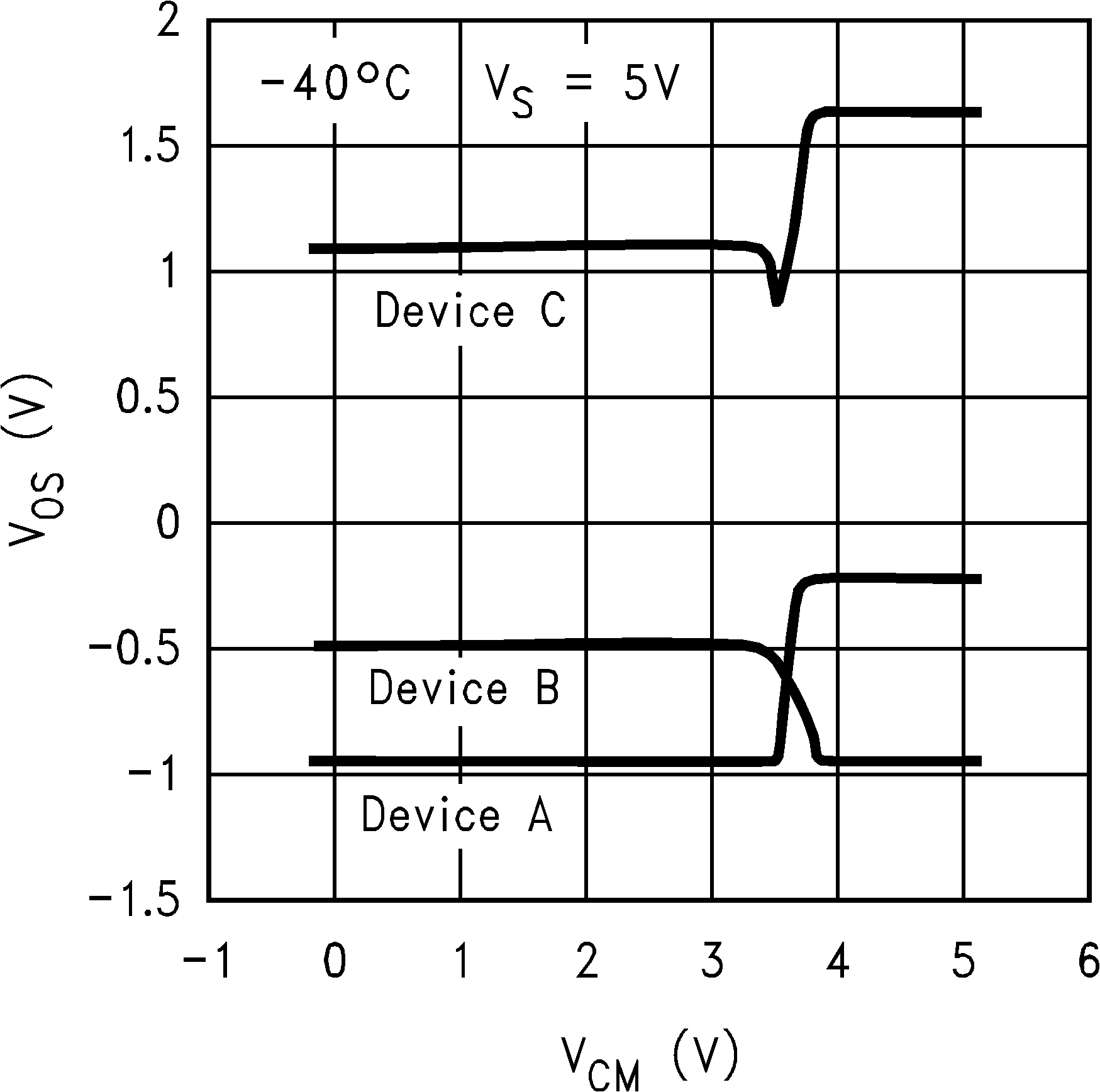Figure 2. VOS vs. VCM for 3 Representative Units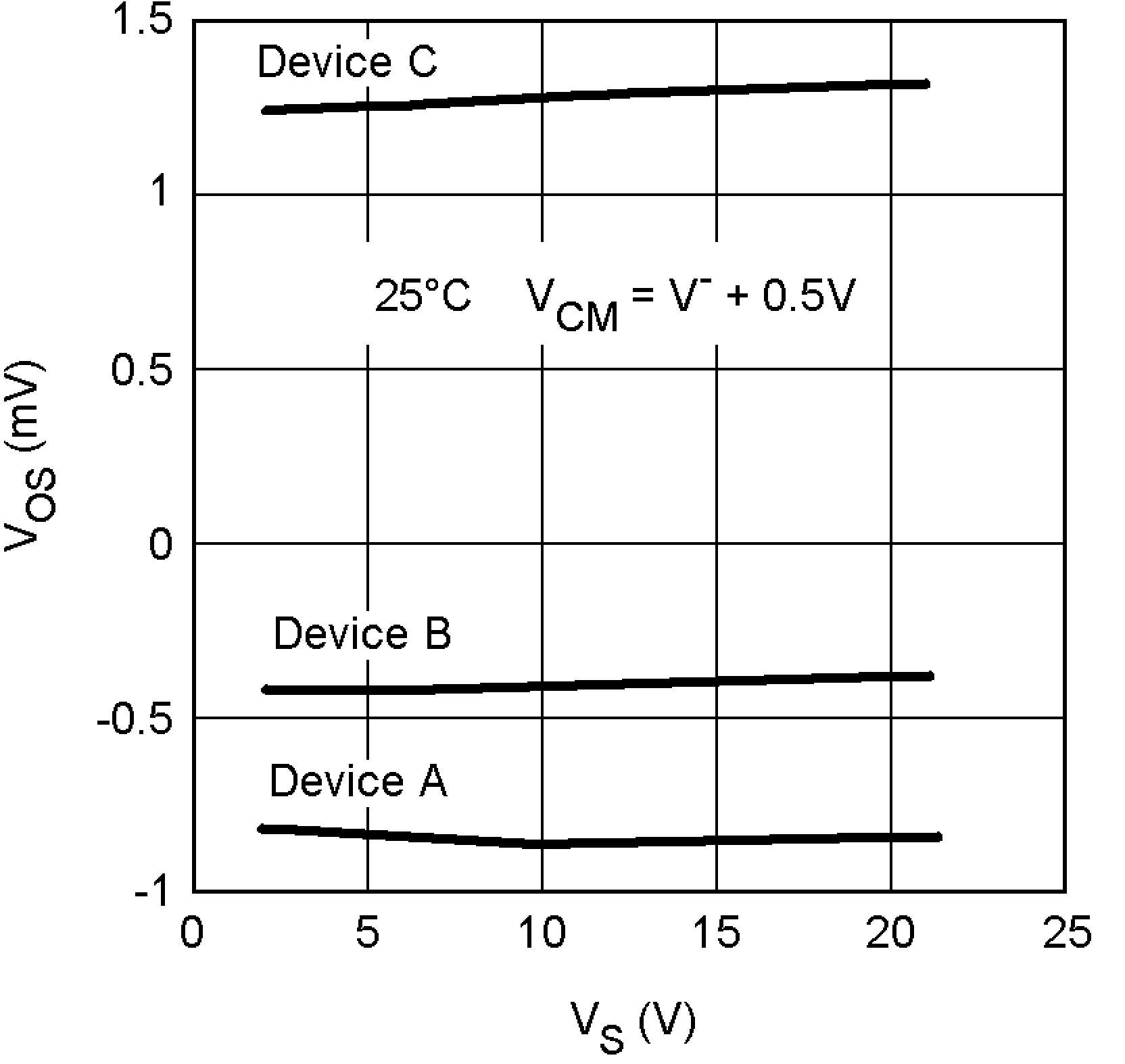Figure 4. VOS vs. VS for 3 Representative Units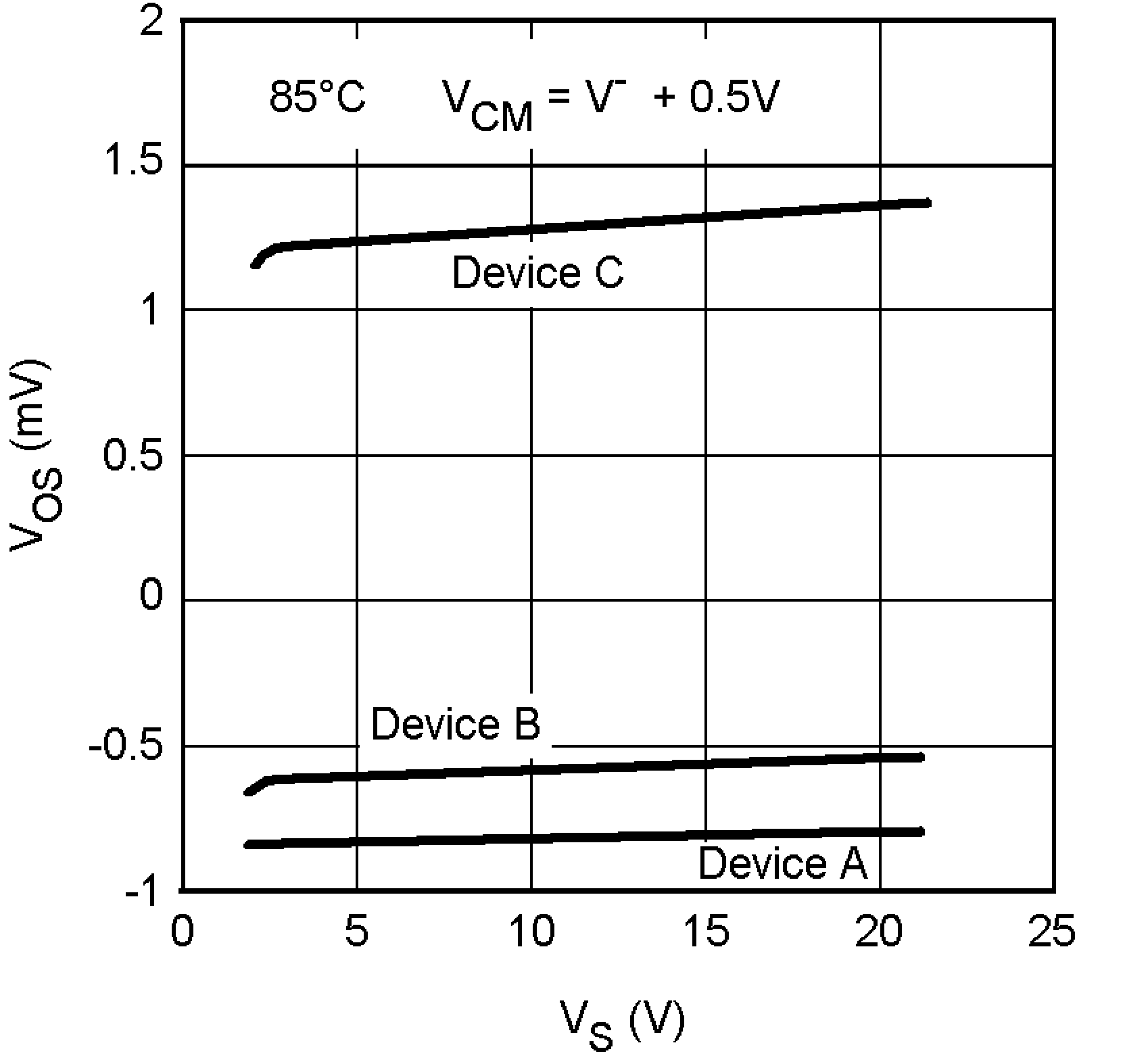Figure 6. VOS vs. VS for 3 Representative UnitsFigure 8. IB vs. VS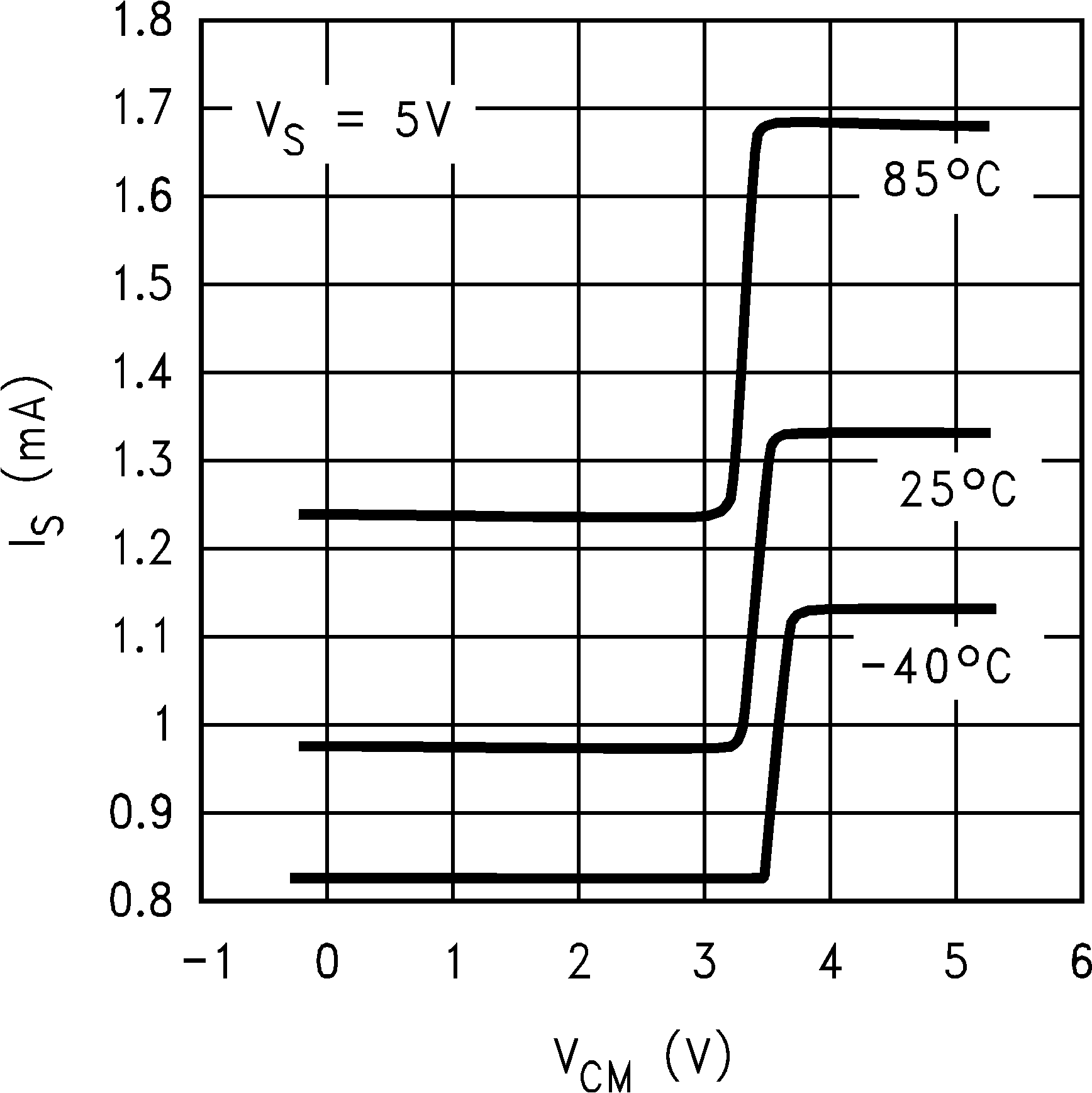Figure 10. IS vs. VCM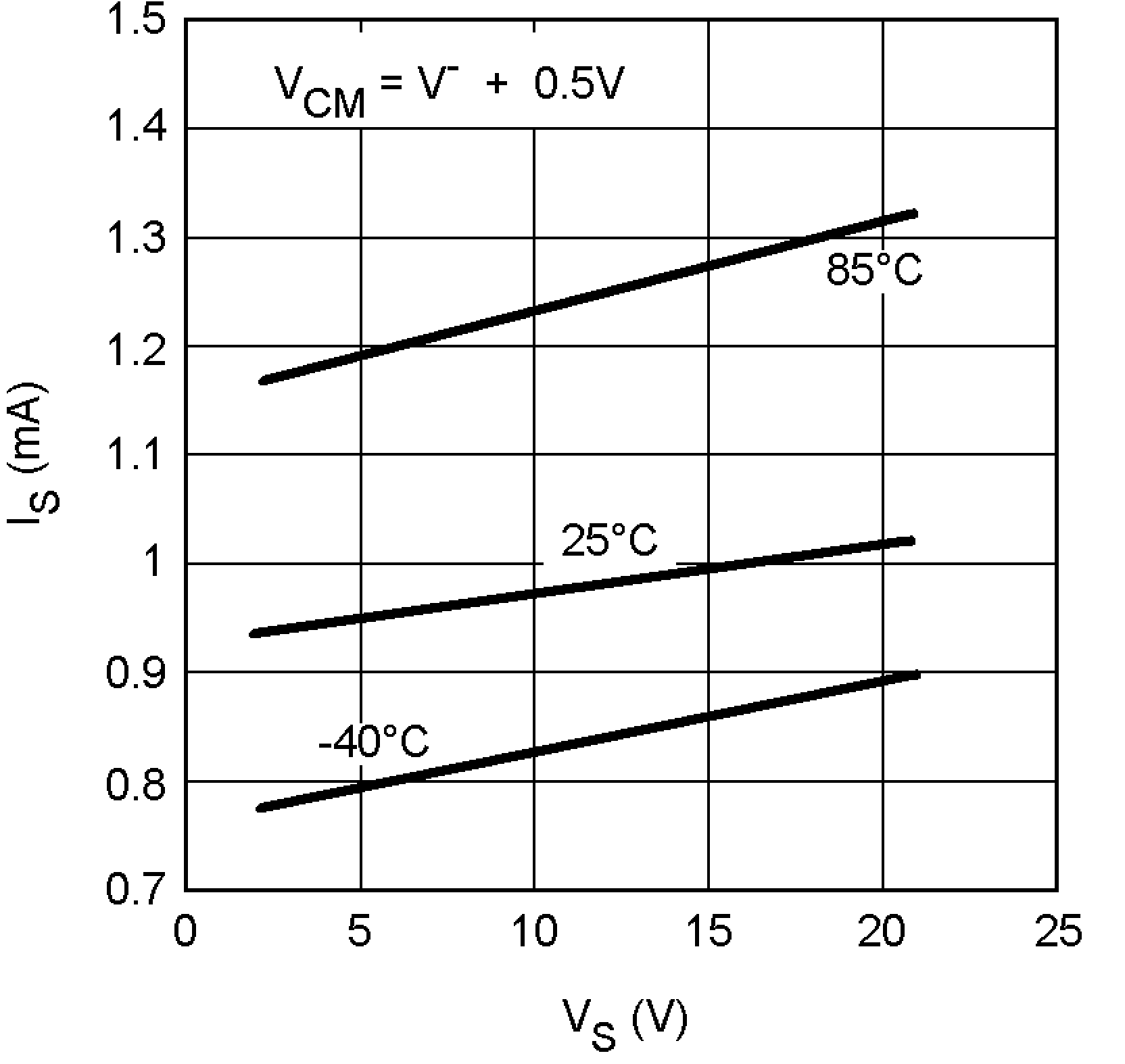Figure 12. IS vs. VS (PNP side)Figure 14. Gain/Phase vs. Frequency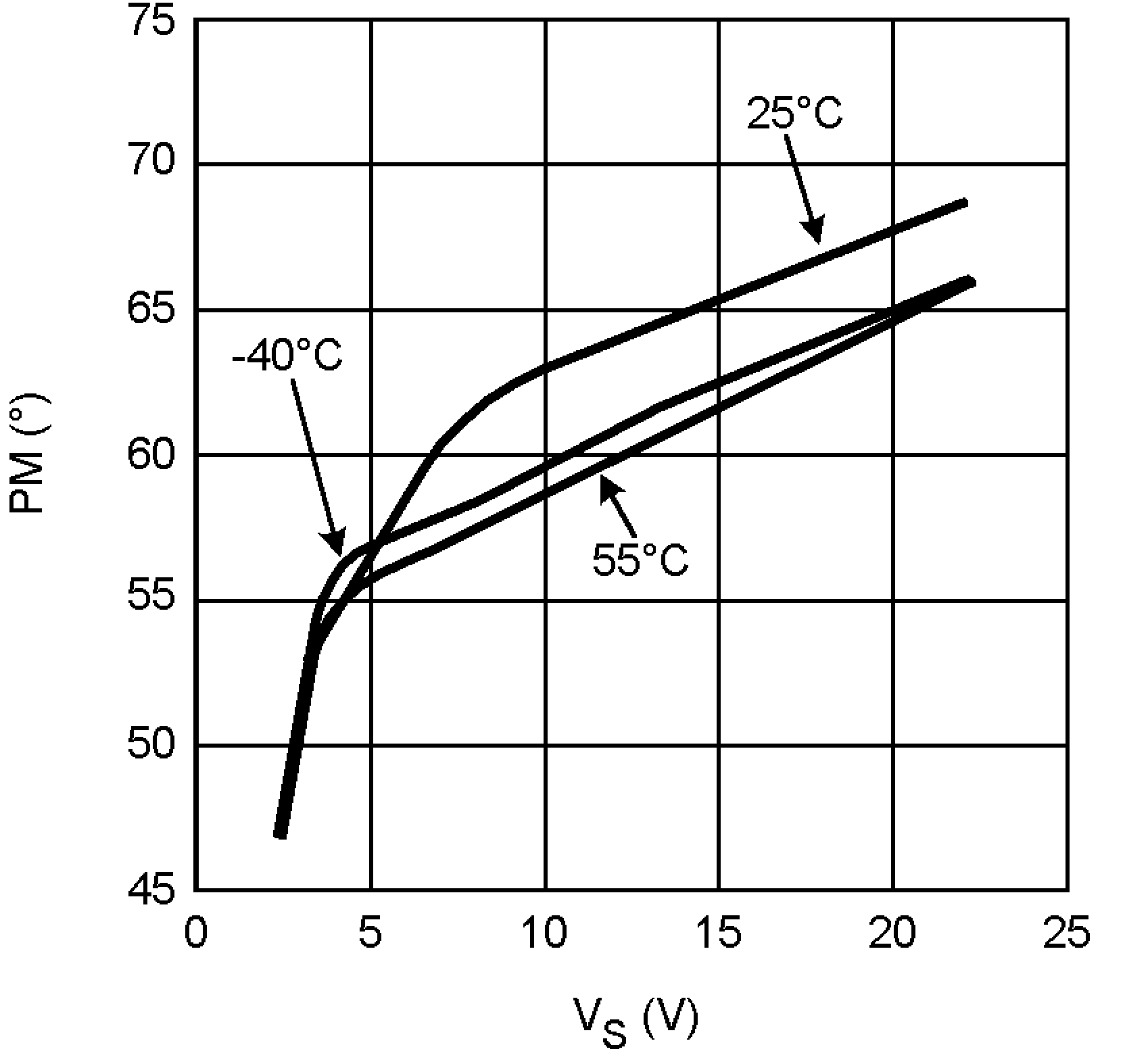Figure 16. Phase Margin vs. VS